图像处理均值化_对于图像的零均值化预处理 - CSDN
• 图像处理中时常会用到图像像素的直方图，但是，一般情况下我们只会使用直方图做为二值化图像的根据，很少会想到处理图像的直方图也可以使图像对比度增加，达到显著的效果。这就是直方图的均值化。 首先，我们从...
在图像处理中时常会用到图像像素的直方图，但是，一般情况下我们只会使用直方图做为二值化图像的根据，很少会想到处理图像的直方图也可以使图像对比度增加，达到显著的效果。这就是直方图的均值化。
首先，我们从图像的直方图说起。什么是直方图？图像直方图，是指对整个图像像在灰度范围内的像素值(0~255)统计出现频率次数，据此生成的直方图，称为图像直方图。直方图反映了图像灰度的分布情况。是图像的统计学特征。
然后，我们再说直方图的均值化。图像中像素个数多的灰度级进行展宽，而对图像中像素个数少的灰度进行压缩，从而扩展像原取值的动态范围，提高了对比度和灰度色调的变化，使图像更加清晰。
最后，用代码的方式演示处理过程：
C++：
#include <opencv2/opencv.hpp>
#include <iostream>
#include <math.h>

using namespace cv;
int main(int argc, char* argv) {
Mat src, dst;
if (src.empty()) {
return -1;
}

cvtColor(src, src, CV_BGR2GRAY);
equalizeHist(src, dst);
char input_title[] = "input image";
char output_title[] = "output image";
namedWindow(input_title, CV_WINDOW_AUTOSIZE);
namedWindow(output_title, CV_WINDOW_AUTOSIZE);

imshow(input_title, src);
imshow(output_title, dst);

waitKey(0);
return 0;
}python：
#! /usr/bin/env python
# -*- coding:utf-8 -*-
import cv2
import matplotlib.pyplot as plt
src = cv2.cvtColor(src, cv2.COLOR_BGR2GRAY)
dst = cv2.equalizeHist(src)
fig = plt.figure(figsize=(15, 10))
plt.imshow(src, "gray")
plt.xticks([]), plt.yticks([]), plt.title("original")
ax2.hist(src.ravel(), 32, [0, 255], color="r")
plt.xticks([0, 255]), plt.title("original_histogram")
plt.imshow(dst, "gray")
plt.xticks([]), plt.yticks([]), plt.title("equalize_result")
ax4.hist(dst.ravel(), 32, [0, 255], color="g")
plt.xticks([0, 255]), plt.title("equalize_histogram")
plt.show()
结果图：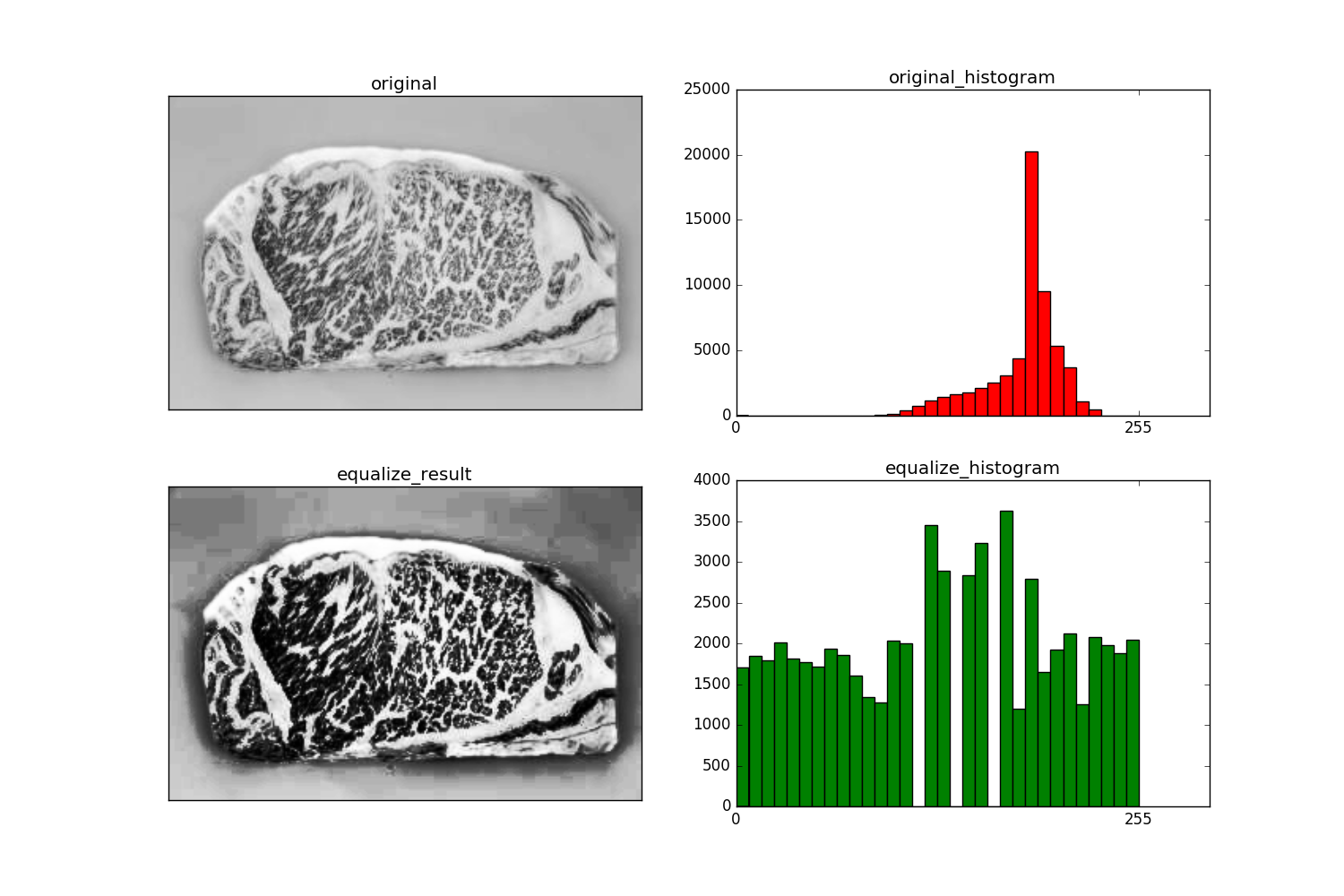可以清楚的看出均值化后的图像比原图像清楚很多，跟其他增强对比度的操作例如掩膜操作，滤波操作等都是差不多的。以后又有一种新的方式可以增强图像的对比度了。
注：
equalizeHist（）函数中输入的图像必须是8-bit的单通道图像。


展开全文图像对比度 python c语言
•  均值滤波是典型的线性滤波算法，它是指在图像上对目标像素给一个模板，该模板包括了其周围的临近像素（如3×3模板：以目标象素为中心的周围8个象素，构成一个滤波模板，即去掉目标象素本身），再用模板中的全体...
1 均值滤波介绍
滤波是滤波是将信号中特定波段频率滤除的操作，是从含有干扰的接收信号中提取有用信号的一种技术。
均值滤波是典型的线性滤波算法，它是指在图像上对目标像素给一个模板，该模板包括了其周围的临近像素（如3×3模板：以目标象素为中心的周围8个象素，构成一个滤波模板，即去掉目标象素本身），再用模板中的全体像素的平均值来代替原来像素值。
均值滤波效果:平滑线性滤波处理降低了图像的“尖锐”变化。由于典型的随机噪声由灰度级的急剧变化组成，因此常见的平滑处理的应用就是降低噪声。均值滤波器的主要应用是去除图像中的不相关细节，其中“不相关”是指与滤波器模板尺寸相比较小的像素区域。然而，由于图像的边缘也是由图像灰度的尖锐变化带来的特性，所以均值滤波处理还是存在着边缘模糊的负面效应。
2 均值滤波算法实现(C语言)1 // junzhilvbo.cpp : 定义控制台应用程序的入口点。
2 //
3
4 #include "stdafx.h"
5 #include "stdlib.h"
6 #include "string.h"
7
8 #define DATA_X 256      //数字图像水平像素个数
9 #define DATA_Y 256      //数字图像竖直像素个数
10
11 void OpenFile(const char *cFilePath , int nOriginalData[DATA_Y][DATA_X])
12 {
13     printf("正在获取数据......\n");
14     FILE *fp ;
15     fp = fopen(cFilePath , "r");
16     if(NULL == fp)
17     {
18         printf("open file failed! \n");
19         return ;
20     }
21
22     unsigned char *pData = (unsigned char *)malloc(sizeof(unsigned char)*DATA_X*DATA_Y);
23     if(NULL == pData)
24     {
25         printf("memory malloc failed!\n");
26         return ;
27     }
28
29     fread(pData , sizeof(unsigned char)*DATA_X*DATA_Y , 1 , fp);
30
31     int count_x = 0 ;
32     int count_y = 0 ;
33
34     for(;count_y < DATA_Y ; count_y++)
35     {
36         for(; count_x < DATA_X ;count_x++)
37         {
38             nOriginalData[count_y][count_x] = pData[count_y*DATA_Y+count_x];
39         }
40     }
41
42     free(pData);
43     fclose(fp);
44
45     return ;
46 }
47
48 void SaveFile(const char *cFilePath , int nResultData[DATA_Y][DATA_X])
49 {
50     printf("正在保存数据......\n");
51     int count_x,count_y;
52
53     FILE *fp ;
54     fp = fopen(cFilePath , "w");
55     if(NULL == fp)
56     {
57         printf("open file failed! \n");
58         return ;
59     }
60
61     for(count_y=0;count_y<DATA_Y;count_y++)
62     {
63         for(count_x=0;count_x<DATA_X;count_x++)
64         {
65             fwrite(&nResultData[count_y][count_x],1,1,fp);
66         }
67     }
68
69     fclose(fp);
70     printf("文件保存成功! \n");
71
72     return ;
73 }
74
75 bool JunZhiLvBo(const int nOriginalData[DATA_Y][DATA_X], int nResultData[DATA_Y][DATA_X])
76 {
77     printf("正在进行均值滤波......\n");
78     int count_x ,count_y ;
79
80     /*3*3模版滤波计算，不计算边缘像素*/
81     for(count_y = 1 ; count_y < DATA_Y ; count_y++)
82     {
83         for(count_x = 1 ; count_x < DATA_X ;count_x++)
84         {
85             nResultData[count_y][count_x] = (int)((nOriginalData[count_y-1][count_x-1]+
86                                                    nOriginalData[count_y-1][count_x]  +
87                                                    nOriginalData[count_y-1][count_x+1]+
88                                                    nOriginalData[count_y][count_x-1]  +
89                                                    nOriginalData[count_y][count_x]    +
90                                                    nOriginalData[count_y][count_x+1]  +
91                                                    nOriginalData[count_y+1][count_x-1]+
92                                                    nOriginalData[count_y+1][count_x]  +
93                                                    nOriginalData[count_y+1][count_x+1])/9);
94         }
95     }
96
97     /*对四个边缘直接进行赋值处理*/
98     for(count_x=0;count_x<DATA_X;count_x++)                                        //水平边缘像素等于原来像素灰度值
99     {
100         nResultData[count_x]=nOriginalData[count_x];
101         nResultData[DATA_Y-1][count_x]=nOriginalData[DATA_Y-1][count_x];
102     }
103     for(count_y=1;count_y<DATA_Y-1;count_y++)                                     //竖直边缘像素等于原来像素灰度值
104     {
105         nResultData[count_y]=nOriginalData[count_y];
106         nResultData[count_y][DATA_X-1]=nOriginalData[count_y][DATA_X-1];
107     }
108
109     return true ;
110 }
111
112 int _tmain(int argc, _TCHAR* argv[])
113 {
114     int nOriginalData[DATA_Y][DATA_X]; //保存原始图像灰度值
115     int nResultData[DATA_Y][DATA_X];   //保存滤波后的灰度值
116
117     memset(nOriginalData,0,sizeof(nOriginalData));  //初始化数组
118     memset(nResultData,0,sizeof(nResultData));
119
120     char cOpenFilePath[] = "Lena.raw";                                           //图像文件路径
121
122     OpenFile(cOpenFilePath,nOriginalData);
123
124     if(!JunZhiLvBo(nOriginalData,nResultData))                                   //滤波计算
125     {
126         printf("操作失败!\n");
127         return 0;
128     }
129
130     char cSaveFilePath[] = "Result.raw";                                        //文件保存路径
131
132     SaveFile(cSaveFilePath,nResultData);
133
134     return 0;
135 }3 均值滤波算法效果对比
均值滤波之前:                                均值滤波之后:展开全文• 为什么要进行图像预处理？大概是为了 （1）使得原始图像符合某种既定规则，便于后续处理。 （2）去除图像中的影响后续处理...大概分为去均值、归一、PCA、白化几种。 假定数据表示成矩阵为X，其中我们假定X是[N...
为什么要进行图像预处理？大概是为了
（1）使得原始图像符合某种既定规则，便于后续处理。
（2）去除图像中的影响后续处理精度、准确度的因素，如噪声等。
（3）在正式处理前进行处理，减少后续的运算量，加速收敛（为什么会提高收敛速度，可以参见这篇博文图像数据预处理对收敛速度的影响），提高后续步骤的可靠性。
大概分为去均值、归一化、PCA、白化几种。

假定数据表示成矩阵为X，其中我们假定X是[N*D]维矩阵，N是样本数据量，D为单张图片的数据向量长度。假设要处理的图像是5*5的彩色图像，那么D即5*5*3=75（因为彩色图像有三个通道），假设N=1000，那么X就是10000*75的矩阵，即1000行图像的信息，每一行代表一个图像的信息。
去均值：
对待训练的每一张图片的特征，都减去全部训练集图片的特征均值，这么做的直观意义就是，我们把输入数据各个维度的数据都中心化到0了（比如数据集3 、5 、7，其均值为5，各减去5后的数据集变为-2、0、2，此时平均值为0）。这么做的目的是减小计算量，把数据从原先的标准坐标系下的一个个向量组成的矩阵，变成以这些向量的均值为原点建立的坐标系，使用python的numpy工具包，这一步可以用X -= np.mean(X, axis = 0)轻松实现。
归一化：
归一化是保证所有的维度上数据都在一个变化幅度上。
比如预测房价的例子中，假设房价由面积s和卧室数b决定，面积s在0~200之间，卧室数b在0~5之间，进行归一化的一个实例就是s=s/2000,b=b/5.
通常我们有两种方法来实现归一化。一个是在数据都去均值之后，每个维度上的数据都除以这个维度上数据的标准差(X /= np.std(X, axis = 0))。 另外一种方式是我们除以数据绝对值最大值，如上面的实例，以保证所有的数据归一化后都在-1到1之间。多说一句，其实在任何你觉得各维度幅度变化非常大的数据集上，你都 可以考虑归一化处理。不过对于图像而言，其实这一步反倒可做可不做，像素的值变化区间都在[0,255]之间，所以其实图像输入数据天生幅度就是一致的。
PCA和白化：
展开全文• matlab语言实现图像处理均值滤波，基于3*3模板，对于初学图像处理者很有帮助，该方法算法简单易懂。均值滤波
• 转自：openCV之中值滤波&amp;均值滤波（及代码实现）：...均值滤波（及代码实现）首先我们看一下图像滤波的概念。图像滤波，即在尽量保留图像细节特征的条件下对目标图像的噪声进行抑...
转自：openCV之中值滤波&均值滤波（及代码实现）：https://blog.csdn.net/weixin_37720172/article/details/72627543

在开始我们今天的博客之前，我们需要先了解一下什么是滤波：

openCV之中值滤波&均值滤波（及代码实现）首先我们看一下图像滤波的概念。图像滤波，即在尽量保留图像细节特征的条件下对目标图像的噪声进行抑制，是图像预处理中不可缺少的操作，其处理效果的好坏将直接影响到后续图像处理和分析的有效性和可靠性。

下图左边是原图右边是噪声图：消除图像中的噪声成分叫作图像的平滑化或滤波操作。信号或图像的能量大部分集中在幅度谱的低频和中频段是很常见的，而在较高频段，感兴趣的信息经常被噪声淹没。因此一个能降低高频成分幅度的滤波器就能够减弱噪声的影响。
图像滤波的目的有两个:一是抽出对象的特征作为图像识别的特征模式;另一个是为适应图像处理的要求，消除图像数字化时所混入的噪声。
而对滤波处理的要求也有两条:一是不能损坏图像的轮廓及边缘等重要信息;二是使图像清晰视觉效果好。
平滑滤波是低频增强的空间域滤波技术。它的目的有两类：一类是模糊；另一类是消除噪音。
空间域的平滑滤波一般采用简单平均法进行，就是求邻近像元点的平均亮度值。邻域的大小与平滑的效果直接相关，邻域越大平滑的效果越好，但邻域过大，平滑会使边缘信息损失的越大，从而使输出的图像变得模糊，因此需合理选择邻域的大小。
关于滤波器，一种形象的比喻法是：我们可以把滤波器想象成一个包含加权系数的窗口，当使用这个滤波器平滑处理图像时，就把这个窗口放到图像之上，透过这个窗口来看我们得到的图像。

举一个滤波在我们生活中的应用：美颜的磨皮功能。如果将我们脸上坑坑洼洼比作是噪声的话，那么滤波算法就是来取出这些噪声，使我们自拍的皮肤看起来很光滑。

这篇博文会介绍中值滤波以及均值滤波两种算法

一.均值滤波

图片中一个方块区域（一般为3*3）内，中心点的像素为全部点像素值的平均值。均值滤波就是对于整张图片进行以上操作。

我们可以看下图的矩阵进行理解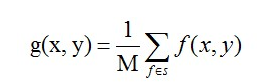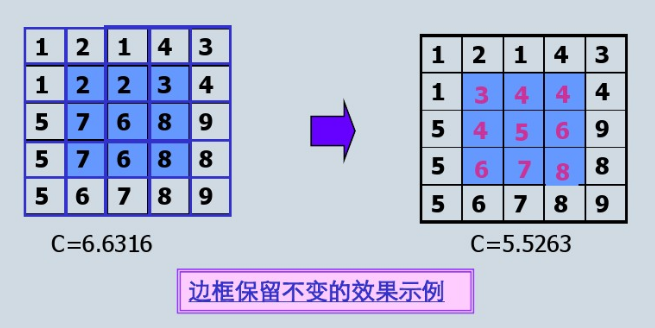缺陷：均值滤波本身存在着固有的缺陷，即它不能很好地保护图像细节，在图像去噪的同时也破坏了图像的细节部分，从而使图像变得模糊，不能很好地去除噪声点。特别是椒盐噪声

实现代码：

#include "opencv2/imgproc.hpp"
#include "opencv2/highgui.hpp"
#include<ctime>
using namespace cv;
using namespace std;

//均值滤波
void AverFiltering(const Mat &src,Mat &dst) {
if (!src.data) return;
//at访问像素点
for (int i = 1; i<src.rows; ++i)
for (int j = 1; j < src.cols; ++j) {
if ((i - 1 >= 0) && (j - 1) >= 0 && (i + 1)<src.rows && (j + 1)<src.cols) {//边缘不进行处理
dst.at<Vec3b>(i, j) = (src.at<Vec3b>(i, j) + src.at<Vec3b>(i - 1, j - 1) + src.at<Vec3b>(i - 1, j) + src.at<Vec3b>(i, j - 1) +
src.at<Vec3b>(i - 1, j + 1) + src.at<Vec3b>(i + 1, j - 1) + src.at<Vec3b>(i + 1, j + 1) + src.at<Vec3b>(i, j + 1) +
src.at<Vec3b>(i + 1, j)) / 9;
dst.at<Vec3b>(i, j) = (src.at<Vec3b>(i, j) + src.at<Vec3b>(i - 1, j - 1) + src.at<Vec3b>(i - 1, j) + src.at<Vec3b>(i, j - 1) +
src.at<Vec3b>(i - 1, j + 1) + src.at<Vec3b>(i + 1, j - 1) + src.at<Vec3b>(i + 1, j + 1) + src.at<Vec3b>(i, j + 1) +
src.at<Vec3b>(i + 1, j)) / 9;
dst.at<Vec3b>(i, j) = (src.at<Vec3b>(i, j) + src.at<Vec3b>(i - 1, j - 1) + src.at<Vec3b>(i - 1, j) + src.at<Vec3b>(i, j - 1) +
src.at<Vec3b>(i - 1, j + 1) + src.at<Vec3b>(i + 1, j - 1) + src.at<Vec3b>(i + 1, j + 1) + src.at<Vec3b>(i, j + 1) +
src.at<Vec3b>(i + 1, j)) / 9;
}
else {//边缘赋值
dst.at<Vec3b>(i, j) = src.at<Vec3b>(i, j);
dst.at<Vec3b>(i, j) = src.at<Vec3b>(i, j);
dst.at<Vec3b>(i, j) = src.at<Vec3b>(i, j);
}
}
}
//图像椒盐化
void salt(Mat &image, int num) {
if (!image.data) return;//防止传入空图
int i, j;
srand(time(NULL));
for (int x = 0; x < num; ++x) {
i = rand() % image.rows;
j = rand() % image.cols;
image.at<Vec3b>(i, j) = 255;
image.at<Vec3b>(i, j) = 255;
image.at<Vec3b>(i, j) = 255;
}
}
void main() {

Mat Salt_Image;
image.copyTo(Salt_Image);
salt(Salt_Image, 3000);

Mat image1(image.size(), image.type());
Mat image2;
AverFiltering(Salt_Image, image1);
blur(Salt_Image, image2, Size(3, 3));//openCV库自带的均值滤波函数
imshow("原图", image);
imshow("自定义均值滤波", image1);
imshow("openCV自带的均值滤波", image2);
waitKey();
}


效果图：可以看到图片变模糊而且噪声并没有很有效的去除，该算法只是模糊化了图片而已。

二.中值滤波

首先，我们复习中值。在一连串数字｛1，4，6，8，9｝中，数字6就是这串数字的中值。由此我们可以应用到图像处理中。依然我们在图像中去3*3的矩阵，里面有9个像素点，我们将9个像素进行排序，最后将这个矩阵的中心点赋值为这九个像素的中值。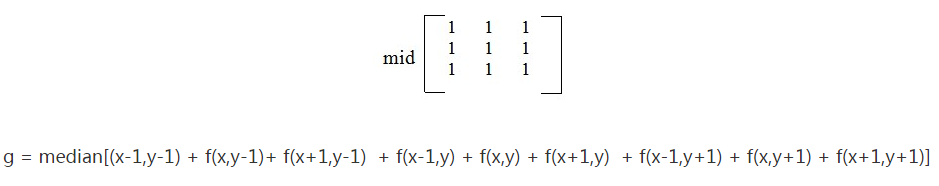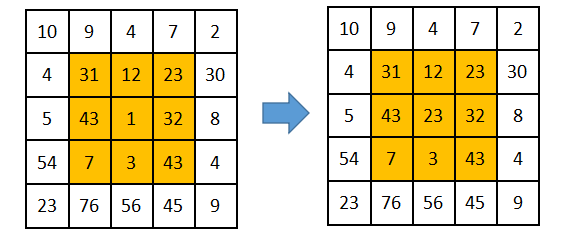代码：

//求九个数的中值
uchar Median(uchar n1, uchar n2, uchar n3, uchar n4, uchar n5,
uchar n6, uchar n7, uchar n8, uchar n9) {
uchar arr;
arr = n1;
arr = n2;
arr = n3;
arr = n4;
arr = n5;
arr = n6;
arr = n7;
arr = n8;
arr = n9;
for (int gap = 9 / 2; gap > 0; gap /= 2)//希尔排序
for (int i = gap; i < 9; ++i)
for (int j = i - gap; j >= 0 && arr[j] > arr[j + gap]; j -= gap)
swap(arr[j], arr[j + gap]);
return arr;//返回中值
}

//图像椒盐化
void salt(Mat &image, int num) {
if (!image.data) return;//防止传入空图
int i, j;
srand(time(NULL));
for (int x = 0; x < num; ++x) {
i = rand() % image.rows;
j = rand() % image.cols;
image.at<Vec3b>(i, j) = 255;
image.at<Vec3b>(i, j) = 255;
image.at<Vec3b>(i, j) = 255;
}
}

//中值滤波函数
void MedianFlitering(const Mat &src, Mat &dst) {
if (!src.data)return;
Mat _dst(src.size(), src.type());
for(int i=0;i<src.rows;++i)
for (int j=0; j < src.cols; ++j) {
if ((i - 1) > 0 && (i + 1) < src.rows && (j - 1) > 0 && (j + 1) < src.cols) {
_dst.at<Vec3b>(i, j) = Median(src.at<Vec3b>(i, j), src.at<Vec3b>(i + 1, j + 1),
src.at<Vec3b>(i + 1, j), src.at<Vec3b>(i, j + 1), src.at<Vec3b>(i + 1, j - 1),
src.at<Vec3b>(i - 1, j + 1), src.at<Vec3b>(i - 1, j), src.at<Vec3b>(i, j - 1),
src.at<Vec3b>(i - 1, j - 1));
_dst.at<Vec3b>(i, j) = Median(src.at<Vec3b>(i, j), src.at<Vec3b>(i + 1, j + 1),
src.at<Vec3b>(i + 1, j), src.at<Vec3b>(i, j + 1), src.at<Vec3b>(i + 1, j - 1),
src.at<Vec3b>(i - 1, j + 1), src.at<Vec3b>(i - 1, j), src.at<Vec3b>(i, j - 1),
src.at<Vec3b>(i - 1, j - 1));
_dst.at<Vec3b>(i, j) = Median(src.at<Vec3b>(i, j), src.at<Vec3b>(i + 1, j + 1),
src.at<Vec3b>(i + 1, j), src.at<Vec3b>(i, j + 1), src.at<Vec3b>(i + 1, j - 1),
src.at<Vec3b>(i - 1, j + 1), src.at<Vec3b>(i - 1, j), src.at<Vec3b>(i, j - 1),
src.at<Vec3b>(i - 1, j - 1));
}
else
_dst.at<Vec3b>(i, j) = src.at<Vec3b>(i, j);
}
_dst.copyTo(dst);//拷贝
}

void main() {

Mat Salt_Image;
image.copyTo(Salt_Image);
salt(Salt_Image, 3000);

Mat image3, image4;
MedianFlitering(Salt_Image, image3);
medianBlur(Salt_Image, image4, 3);
imshow("自定义中值滤波处理后", image3);
imshow("openCV自带的中值滤波", image4);
waitKey();
}


效果图：可以看到，椒盐噪声很好的被平滑了，而且也没均值那样模糊化太过于严重。

三 填充问题

在对图像应用滤波器进行过滤时，边界问题是一个需要处理的问题。一般来说，有3种处理的方法。

1. 不做边界处理

不对图像的边界作任何处理，在对图像进行滤波时，滤波器没有作用到图像的四周，因此图像的四周没有发生改变。

2. 填充0

对图像的边界做扩展，在扩展边界中填充0，对于边长为2k+1的方形滤波器，扩展的边界大小为k，若原来的图像为[m, n]，则扩展后图像变为[m+2k, n+2k]。进行滤波之后，图像会出现一条黑色的边框。

3. 填充最近像素值

扩展与 填充0 的扩展类似，只不过填充0的扩展是在扩展部分填充0，而这个方法是填充距离最近的像素的值。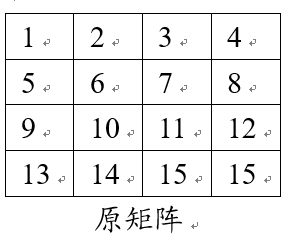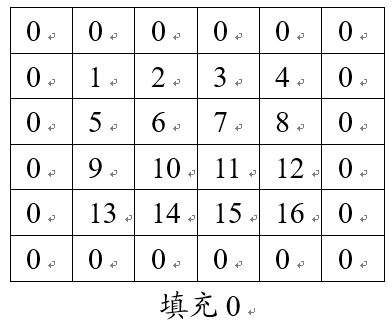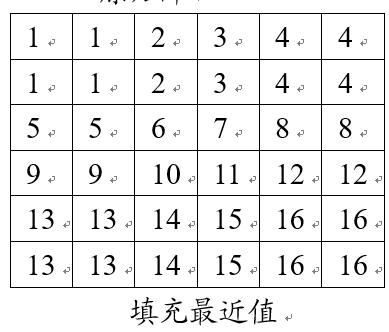四 总结：

均值滤波和和中值滤波都可以起到平滑图像，虑去噪声的功能。

均值滤波采用线性的方法，平均整个窗口范围内的像素值，均值滤波本身存在着固有的缺陷，即它不能很好地保护图像细节，在图像去噪的同时也破坏了图像的细节部分，从而使图像变得模糊，不能很好地去除噪声点。均值滤波对高斯噪声表现较好，对椒盐噪声表现较差。

中值滤波采用非线性的方法，它在平滑脉冲噪声方面非常有效,同时它可以保护图像尖锐的边缘，选择适当的点来替代污染点的值，所以处理效果好，对椒盐噪声表现较好，对高斯噪声表现较差。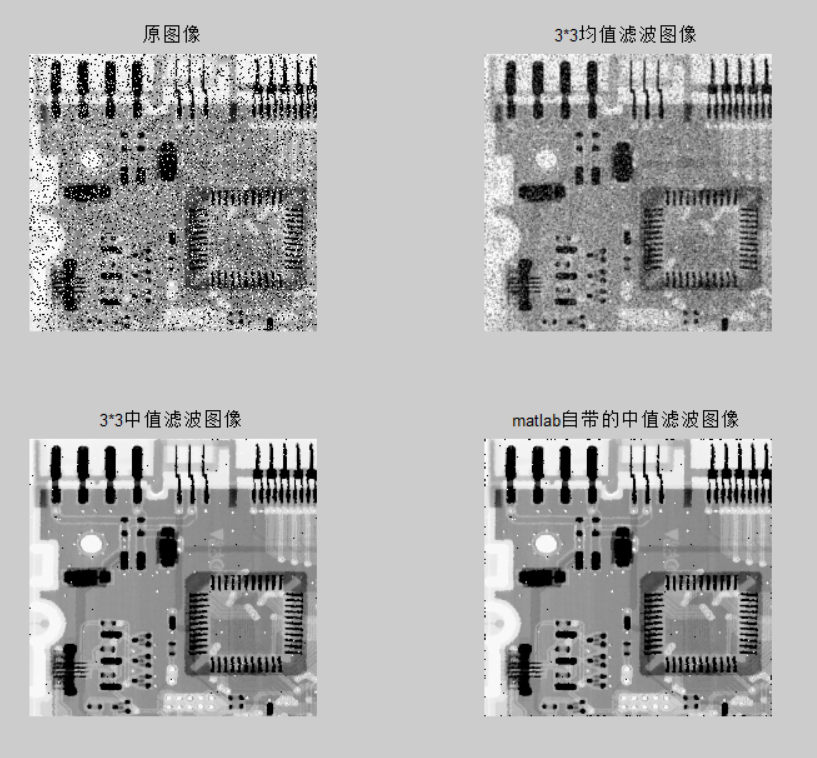参考：

1.均值滤波和中值滤波

https://blog.csdn.net/cjsh_123456/article/details/79261271

2.
展开全文图像处理 中值滤波 均值滤波
• 直方图均值化实验简述实验要求： 下载一曝光不足和一曝光过度的彩色图片，对彩色图片进行直方图均衡化，并保存和展示。原理简述直方图概念 直方图（Histogram）又称质量分布图、柱状图，是一种统计报告图，也是...opencv 图像处理
• 神经网络图像输入零均值化的作用 最近有和同学探讨到为什么CNN在做图像识别相关任务时，对图像需要进行零均值化，CS231N里面有进行讲述，但讲得其实不是很清楚，比较难理解，所以在这里做一下较为详细的说明。 1 ...深度学习 卷积神经网络 数据预处理 图像
• 版权声明：本文为博主原创文章，未经博主允许不得转载。... ... 图像处理之积分图应用四（基于局部均值的图像二值算法） 基本原理 均值法，选择的阈值是局部范围内像...
• 本系列博客，注重图像处理领域基本算法的原理，主要用matlab语言实现。 1、滤波模板的产生 首先，滤波要有模板，在matlab中。得到模板的途径主要有两种，一种是自己定义，另一种是通过调用函数fspecial得到各种...均值滤波 非线性滤波 模板
• 均值滤波器中，模板内像素的权重都为一，其只是简单的像素加法平均 而，加权均值滤波器，对模板中的像素点赋予不同的权重，求的是像素的加权平均，典型的模板，例如高斯模糊，其模板权重呈现钟型的高斯分布： ...数字图像处理 加权均值滤波 Python
• opencv中图像均值化都是基于灰度图的。 直方图的均值化能提高图片的对比度。  下面介绍两种方法 自带函数均值化 cv.equalizeHist() 参数： 输入一个8比特的单通道图像 自定义均值化 上述的直方图均衡化...
• 一....缺陷：均值滤波本身存在着固有的缺陷，即它不能很好地保护图像细节，在图像去噪的同时也破坏了图像的细节部分，从而使图像变得模糊，不能很好地去除噪声点。 原图： 对比图： ...数字图像处理 滤波
• 一、中心化（又叫零均值化）和标准化（又叫归一化）概念及目的？1、在回归问题和一些机器学习算法中，以及训练神经网络的过程中，通常需要对原始数据进行中心化（Zero-centered或者Mean-subtraction（subtraction...
• 在机器学习回归问题，以及训练神经网络过程中，通常需要对原始数据进行中心化（零均值化）与标准化（归一化）预处理。 目的：通过中心化和标准化处理，最终得到均值为0，标准差为1的服从标准正态分布的数据。 原理...数据预处理 归一化
• 基于MATLAB图像处理的中值滤波、均值滤波以及高斯滤波的实现与对比 1.背景知识 中值滤波法是一种非线性平滑技术，它将每一像素点的灰度值设置为该点某邻域窗口内的所有像素点灰度值的中值. 中值滤波是基于排序...matlab 图像处理 算法 高斯滤波 中值滤波
• 在训练神经网络的过程中，通常需要对原始数据进行中心（Zero-centered或者Mean-subtraction（subtraction表示减去））处理和标准（Standardization或Normalization）处理。下面，我将介绍这两个操作的作用。 零...深度学习
• 归一 进行归一的原因是把各个特征的尺度控制在相同的范围内，这样可以便于找到最优解，不进行归一时如左图，进行归一后如右图，可...另一种是均值方差归一，一般是把均值归一成0，方差归一成1。适用于...归一化
•均值滤波
• 该系列文章是讲解Python OpenCV图像处理知识，前期主要讲解图像入门、OpenCV基础用法，中期讲解图像处理的各种算法，包括图像锐化算子、图像增强技术、图像分割等，后期结合深度学习研究图像识别、图像分类应用。...Python 图像处理 OpenCV 阈值化处理 threshold
...